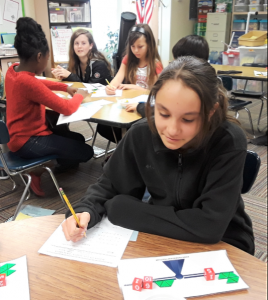# Hands-On Equations Fractions Class Sets

Leave a Review

\$89.50\$209.50

Includes The Hands-On Equations Fractions Learning System for the teacher and a Hands-On Equations Fractions Student Kit for each student. Classroom worksheets with reproduction rights, and an answer key are included.

SKU: N/A

## Description

### Methodology

This Hands-On Equations Fractions program consists of two levels encompassing a total of 12 lessons. As with the original Hands-On Equations program, students represent the fractional equation on a flat laminated scale using their game pieces, and then use “legal moves” to solve the equations. Each lesson comes with a worksheet containing 8 problems.

Unique to the Hands-On Equations Fractions approach, students solve first for the unit fraction of the unknown. Hence, for example, if the blue block representing a third of the unknown y is found to have a value of 4, they can figure out that the yellow block, representing the unknown y, has a value of 12.  This methodology provides students with a sense-making approach to fractional linear equations.

Each level ends with an abstract solution approach which may be presented to younger students as an enrichment lesson. For middle or high school students, it is important that the teacher lead them to solutions using only paper and pencil.

### Materials needed for each student

Each student needs the Hands-On Equations Fractions Student Kit. This kit consists of a set of 8 numbered cubes, a flat laminated scale, and a set of 33 plastic fraction blocks, and a white plastic bowl.